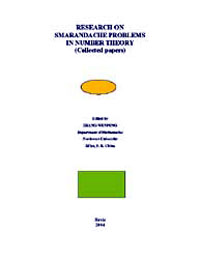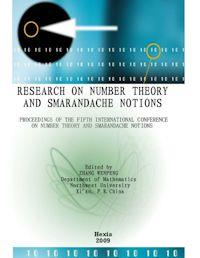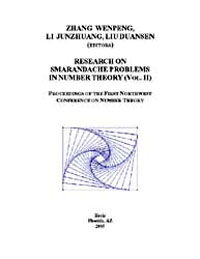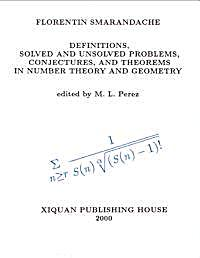#jsDisabledContent { display:none; } My Account | Register | Help

ArithmeticArithmetic or arithmetics (from the Greek word ????µ?? = number) is the oldest and most elementary branch of mathematics, used by almost everyone, for tasks ranging from simple day-to-day counting to advanced science and business calculations. It involves the study of quantity, especially as the result of combining numbers. In common usage, it refers to the simpler properties when using the traditional operations of addition, subtraction, multiplication and division with smaller values of numbers. Professional mathematicians sometimes use the term (higher) arithmetic when referring to more advanced results related to number theory, but this should not be confused with elementary arithmetic.•A Key to Dilworth's Arithmetic; Wherein Every Question Is Worked O...

By: A Teacher of Arithmetic in New York

The original plan included 6 pt. in 3 v. Only pt. 1-2, v.1 and pt. 6 [v.3, pt. 2] were ever published.-cf. L.C. Catalog.

•First Book in Arithmetic : Comprising Two Years of Oral and Writte...

By: Harpers' Graded Arithmetics, Harper, Firm, Publishers, New York, Harper & Brothers
•First Book in Arithmetic : Comprising Two Years of Oral and Writte...

By: Harpers' Graded Arithmetics, Harper, Firm, Publishers, New York, Harper & Brothers
•Teacher's Book of Mental Arithmetic. [7 Pt. In 1 Volume ]

By: Mental Arithmetic
•Complete Arithmetic : Comprising the New Intermediate Arithmetic a...

By: Stoddard A. Felter, Samuel Ashbel Farrand
•Teacher's book of mental arithmetic. [7 pt. in 1 vol.]

By: Mental Arithmetic
•Introduction to Number Theory

By: George E. Andrews

Description: Although mathematics majors are usually conversant with number theory by the time they have completed a course in abstract algebra, other undergraduates, especially those in education and the liberal arts, often need a more basic introduction to the topic. In this book the author solves the problem of maintaining the interest of students at both levels by offering a combinatorial approach to elementary number theory. In studying number theory from such a per...

•Research on Smarandache Problems in Number Theory

By: Zhang Wenpeg, Editor

Number theory is an ancient subject, but we still cannot answer many simplest and most natural questions about the integers. Some old problems have been solved, but more arise. All the research for these ancient or new problems implicated and are still promoting the development of number theory and mathematics.

•Research on Number Theory and Smarandache Notions : Proceedings of...

By: Florentin Smarandache; Zhang Wenpeng, Editor

This book contains 23 papers, most of which were written by participants to the fifth International Conference on Number Theory and Smarandache Notions held in Shangluo University, China, in March, 2009. In this Conference, several professors gave a talk on Smarandache Notions and many participants lectured on them both extensively and intensively. All these papers are original and have been refereed. The themes of these papers range from the mean value or hybrid mean va...

3. Remarks Sandor  has considered the problem of finding the S-perfect and completely S-perfect numbers, but his proof is not complete. He has proved that the only S-perfect of the form n = p q is n = 6 and there is no S-perfect number of the form n = 2kq where k ¸ 2 is an integer and q is an odd prime. On the other hand, Theorem 2.1 gives all the S-perfect numbers. Again, Sandor only proved that, the only completely S-perfect number of the form n = p2q is n = 28, an...

•Algebra and Number Theory

By: A. Baker
•Research on Smarandache Problems in Number Theory (Volume Ii)

By: Zhang Wenpeg, Li Junzhuang, Liu Duanses

For any positive integer n, let (n) be the Euler function, and am(n) denotes the Smarandache m-th power residues function of n. The main purpose of this paper is using the elementary method to study the number of the solutions of the equation (n) = am(n), and give all solutions for this equation.

•Lectures on Topics in Algebraic Number Theory

By: Sudhir R. Ghorpade

Description: These are the notes of a course of ten lectures given at the Christian-Alberchts-Universität Kiel at Kiel, Germany during December 2000. These lectures were aimed at giving a rapid introduction to some basic aspects of Algebraic Number Theory with as few prerequisites as possible. The Table of Contents below gives some idea of the topics covered in these notes. There are two appendices, first containing author's older notes on Galois Theory and the second re...

•Introductory Number Theory

By: Michael Stoll
•Algebraic Number Theory

By: Technical Books Center
•Number Theory

By: Technical Books Center
•A Course on Number Theory

By: Peter J. Cameron
•Number Theory: Fermats Last Theorem

By: Simon Singh

Description: Fermat's Last Theorem is a popular science book by Simon Singh. It tells the story of the search for a proof of Fermat's last theorem, first conjectured by Pierre de Fermat in 1637. Despite the efforts of many mathematicians, the proof would remain incomplete until as late as 1995, with the publication of Andrew Wiles' proof of the Theorem.

•Definitions, Solved and Unsolved Problems, Conjectures, and Theore...

By: Florentin Smarandache; M L. Perez, Editor

Florentin Smarandache, an American mathematician of Romanian descent has generated a vast variety of mathematical problems. Some problems are easy, others medium, but many are interesting or unsolved and this is the reason why the present book appears. Here, of course, there are problems from various types. Solving these problems is addictive like eating pumpkin seed: having once started, one cannot help doing it over and over again.

•Elementary Arithmetic on the Unitary System [microform] : Intended...

By: Smith, J. Hamblin (James Hamblin), 1829-1901. a Treatise on Arithmetic

Description: Tables

•Turnpike theorems for integer programming problems

By: Shapiro, Jeremy F., 1939

Supplemental catalog subcollection information: American Libraries Collection; American University Library Collection; Historical Literature; Bibliography: leafCopyright © World Library Foundation. All rights reserved. eBooks from iCloud eBook Library are sponsored by the World Library Foundation,
a 501c(4) Member's Support Non-Profit Organization, and is NOT affiliated with any governmental agency or department.